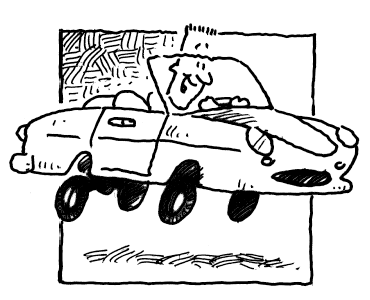### Home > PC3 > Chapter 13 > Lesson 13.3.1 > Problem13-105

13-105.Sam accelerates his car according to the formula $v\left(t\right) = 6t^{2}$, where $t$ is the time in seconds and $v\left(t\right)$ is velocity in ft/s.

1. Sketch a velocity vs. time graph for the first two seconds.

2. How can the graph be used to approximate distance?

3. Jake approximated the distance traveled by the car during the first two seconds by writing distance $=\left(0.1\right)[6\left(0.1\right)^2+$$6\left(0.2\right)^2+6\left(0.3\right)^2$$+\dots+6\left(2\right)^2]$. Explain what he is doing. Include as much detail as you can.

4. Rewrite Jake’s distance equation in part (c) in sigma notation.

5. Margaret approximated the distance traveled by the car during the first two seconds by using midpoint rectangles. Write Margaret’s sum in sigma notation if she used the same number of rectangles as Jake.

The endpoints are now $0.05, 0.15, 0.25$, etc. Write an expression for this sequence.

6. Use your area program to evaluate Jake’s sum and approximate the distance traveled.